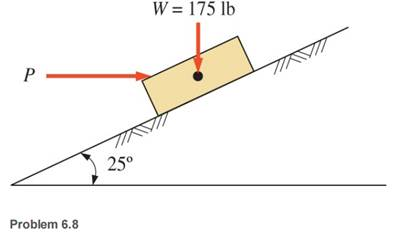### Create an Account

Home / Questions / Compute the horizontal force P required to prevent the block from sliding down the plane f...

# Compute the horizontal force P required to prevent the block from sliding down the plane for the 175 lb block shown Assume the coefficient of static friction to be

Compute the horizontal force P required to prevent the block from sliding down the plane for the 175 lb block shown. Assume the coefficient of static friction to be 0.65Jun 26 2020 View more View LessSubscribe To Get Solution